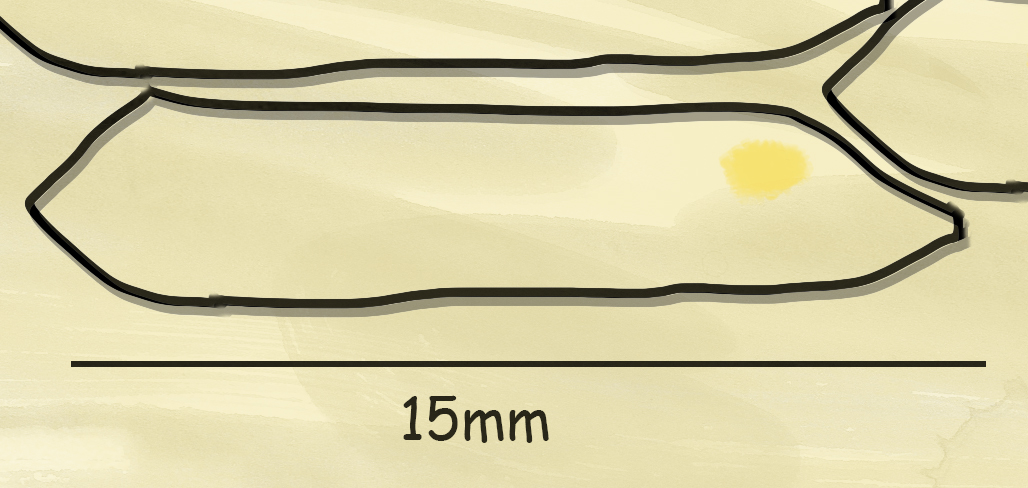# Magnification

After looking at cells under a microscope you may be asked to do a few calculations. These can be tricky but there is a great way to remember how to these calculations.

Below is the triangle you can use to help you learn and remember with the 3 equations needed. Simply remember it as AIM!!

Using the triangle is just as easy. Simply cover the one you want to work out and if they are next to each-other then you multiply and if they are on top/below each together then you need to divide to get your answer.Actual image is the real size of the “thing” you are looking at (sometimes called The Object).

Image Size is the measurement, length/height/width, of the image you are looking at.

Magnification is how many times bigger something is being observed compared to its actual size.

When looking at cells we are looking at very small things so you may see some new measurements that you need to understand.The common one to remember is that 1mm = 1000µm

Top Tip – For any questions you get it usually helps to convert all your units to the same unit first.

## Example - MagnificationYou are given an image of a cell and you measure the width to be 2mm. You are told that the Actual Image size is 0.02mm so you can now easily work out the magnification using the equation below.Since they are already both in the same units you don’t need to convert them and just put them straight into the equation so you have.

Magnification =  2/0.02 = 100

This means the diagram has been magnified 100 times

## Example - Image Size

This time you have been told that the actual size of what you are looking at is 400µwide and that the magnification is x50We have we need to wok it out and can just substitute into the equation.

Image Size = 0.4 x 50 = 2,000

So the image size is 20,000µm or 20mm

## Example - Actual SizeHere we have an image of a cell. It measures 15mm wide and the magnification is x400.Using the equation we can work out what the real size of the cell is.

Actual Image = 15/400 = 0.0375

So the real cell is 0.0375mm wide. You will want to convert this into µm so just times it by 1000 giving us a width of 37.5µm

Once you can use the equations and convert units with ease you can also use the same process to measure sub-cellular structures in the cells and estimate the surface area etc…